Next: Difficult problems Up: benches Previous: Integration

• La page de J-P. Merlet
• J-P. Merlet home page
• La page Présentation de COPRIN
• COPRIN home page
• La page "Présentation" de l'INRIA
• INRIA home page

Subsections

# Other problems: inequalities, parametric polynomials,..

## Binjia

Origin:jiaob@cas.mcmaster.ca

In this problem we have a polynomialof degree 18 in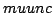and 11 in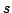and we have to find at least onesuch that one of the real root s ofverify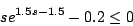We solve this problem as a non 0-dimensional problem: we will find ranges for s,such that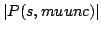is very small and s satisfies the above constraint.

Alternatively we may use our continuation module that will allow to display all the roots s as a function ofand visually select the value ofsuch that the constraint is satisfied.

The polynomialis too large to be displayed here but may be obtained with the following Maple program:


lambda:=0.8;
mu:=1.5;
muack:=0.1;
G:= proc(x,muunc)
(1/muack)^x*(muunc/(muunc-lambda))*sum('(muack/(mu-muunc))^k*(1/(x-k)!)*
(1/(1-lambda/muunc)^k)','k'=0..x)
end:
Pis:=proc(M,k,muunc)
(1/G(M,muunc))*(1/(mu-muunc))^(M-k)*(1/k!)*(1/muack)^k*
(1/(1-lambda/muunc))^(M-k+1)
end:
findsig:=proc(s,M,muunc)
sum('Pis(M,k,muunc)*(lambda+k*muack)/(mu-mu*s+lambda+k*muack)','k'=0..M)
end:
ew:=findsig(s,9,muunc)-s:
ew:=numer(ew):

P:=op(5,ew):

Range:: [-10,10] for all unknowns

Solving method: GeneralSolve
Solutions:: 1 (approximate)
Computation time (April 2003):

 Sun Blade 6.03s

## SchoolBuilding

Origin: COCONUT, taken from SpaceSolver, LIA/EPFL, http://liawww.epfl.ch/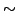lottaz/SpaceSolver/
We have 6 inequalities for 5 unknowns:


.1666666667e-2*u-(.318e-4*H_s+.54e-2)*S+.1e-11 <= 0

137.7-.8633e-1*S+.5511e-4*S^2-.8358e-8*S^3-H_s <= 0

.77e-1*((.1666666667e-2*u+.7783292378e-6*W^1.5161)*W^2)^.3976+.1e-11-H_b <= 0

.168e-1*(S*W^3)^.2839+.1e-11-H_b <= 0

.1666666667e-2*u+.7783292378e-6*W^1.5161-50 <= 0

15-.1666666667e-2*u-.7783292378e-6*W^1.5161 <= 0

The unknowns are:

[H_b, H_s, S, W, u]

Range:: [150, 400], [50, 200], [1500., 2200], [3000, 13500], [6000, 36000]
The original problem is to find one value for each of the unknowns so that the inequalities are satisfied. But with our interval analysis solver we are able to calculate not one value but a set of sets of possible values. Each set is provided as a range for each unknown and any value for the unknowns in this range will satisfy the inequalities. For example we get the set:

[306.9,400],[132.14344,137.53982],[2112.5,2200],
[5625,6937.5],[10397.0999992237,12105.3999984474]

Hence examples of possible value of the unknowns is (307, 133, 2113, 5700, 10398) or (399,136, 2199,600,12000).

Hence we obtain an approximation of the solution variety and furthermore we obtain an information of the quality of this approximation: we may compute the total volume of the approximation and we have also an upper bound of the total volume of the variety. For example we get here that the total volume of the variety volume is 1.20477e+12+ [0,2.66039e+14] or for a better approximation 2.04418e+12+ [0,2.51944e+14]. Furthermore we can adjust the accuracy of the approximation incrementally.

Solving method: GradientSolve +HullIConsistency+ 3B
Computation time (May 2004):

 DELL D400 (1.7Ghz) 1.74s (Vol=1.20477e+12), 5.41s (Vol=2.04418e+12)

## StackedGymnasticHalls

Origin: COCONUT, taken from the PhD Thesis by Claudio Lottaz, LIA/EPFL.
Physical meaning: a civil engineering problem

We have 18 inequalities for 9 unknowns:


P-4.999999999999 <= 0

5.500000000001-1.*H_s1 <= 0

5.500000000001-1.*H_s2 <= 0

2.500000000001-1.*E <= 0

4.5*t-1.*c <= 0

c-85.*t <= 0

40.*d-1.*h_a <= 0

h_a-100.*d <= 0

252661.8726678*E^1.6*L^4-.220000*h_a^2*(3.37*h_a*d+42.84*t*c) <= 0

3300.00*L*E^(8/5)-67.8386566297810273*h_a*d <= 0

825.00*L*E^(8/5)-.47*t*c*h_a <= 0

H_s1+H_s2+.1e-2*h_a-10.999999999999-1.*P <= 0

100.*E^1.6*L-.1e7 <= 0

-100.*E^1.6*L+1. <= 0

200.*E^.6-2000. <= 0

-200.*E^.6+200. <= 0

.220000*h_a^2*(3.37*h_a*d+42.84*t*c)-.1e9*L^3 <= 0

-.220000*h_a^2*(3.37*h_a*d+42.84*t*c)+L^3 <= 0

The unknowns are:

[E, H_s1, H_s2, L, c, d, h_a, P, t]

and lie in the following ranges:

[2, 10], [5, 8], [5, 8], [10, 50], [200, 500], [20, 40], [800, 4000],
[0, 10], [20, 100]

As in the SchoolBuilding bench we get various solutions for the unknowns. We get almost immediately the following solution:

E=[2.500000000001,2.50000058157186]
H_s1=[5.500000000001,5.50000078503804]
H_s2=[5.500000000001,5.50000078503804]
L=[42.5,43.75]
c=[445.1214375,472.56071875]
d=[36.7682900289095,40]
h_a=[3353.6580057819,3569.10533718793]
P=[4.17682900289195,4.58841450144547]
t=[90,95]

Solving method: GradientSolve +HullIConsistency+ 3B

## SteelBuilding

Origin: COCONUT, taken from the PhD Thesis by Claudio Lottaz, LIA/EPFL.
Physical meaning: a civil engineering problem

Constants:


ZeroPlus= 1e-12         E= 0.21e12          F_r= 0.4
G_m= 1500              G_q= 1.5             G_r= 1.1
L_sc= 4000             M_FH= 3.5            Q_r= 5000
d_o= 0.5e-1            f_y= 2.35e8          t_fs= 0.03
t_s = 0.12             t_w = 0.012

Equalities

L = 2*x + d + (n_h - 1)*e                 F_H = M_FH + h + t_s + t_fs
B_V = F_H*A_b         h = 2*a + d         h = b + t
N_1*a_N = M           M = (q*L^2)/8       q = (1.3*G_m + G_q*Q_r)*b_s
a_N = S/A_1           S = 2*c*t*b + t_w*(a - t)*(b - a)
A_1 = 2*c*t + t_w*(a - t)                N_1pl = f_y*A_1
T_d = V_1/A_1w        A_1w = (a - t/2)*t_w         V_1 = q/2 * (L/2 - y_1)
y_1 = x + d/2         y_2 = y_1 + e                V_1pl = f_y*A_1w/sqrt(3)
V_2pl = f_y*A_2/sqrt(3)       A_2 = (e - d)*t_w
w_l = 5/384*q_sc*L^4/(E*I_y)             q_sc = L_sc*b_s
I_y = 4*c*t*(b/2)^2 + t_w*(a - t) * (b - a)^2/2            c_n = A_1/(2*t_w)
Z_1 = c_n^2*t_w/2 + (a - c_n - t_w)^2/ 2*t_w + 2*(a - c_n - t/2)*c*t
V_f = n_d*F_r*Pi*d_v^2/4

Inequalities

n_h >= n_d

# Constraints by contractor

x >= 1.5*d + ZeroPlus
e >= 2.5*d + ZeroPlus
d <= 0.75*h - ZeroPlus

# Constraints by civil engineer

n_h >= 3
N_1 <= N_1pl/G_r - ZeroPlus
(N_1/A_1 + V_1*d/4/Z_1)^2+ZeroPlus <= (f_y^2 - 3*T_d^2)/G_r
V_1 <= V_1pl/G_r - ZeroPlus
V_2 <= V_2pl/G_r - ZeroPlus
(a - t/2)/t_w <= 11 - ZeroPlus
1.2*w_l <= L/350 - ZeroPlus
t_w*(a - t) >= 2*c*t + ZeroPlus

# Constraints by Ventilation expert

d >= d_v + d_o + ZeroPlus
V_f >= c_V*B_V + ZeroPlus

The range for the unknowns are defined by

# 42 variables

A_1    >=1e-5  ,<=0.16    #8.36e-3 chord area
A_1w  >=0  ,<=0.08        #1.6e-3 web area of chord
A_2    >=0  ,<=1.2        #6.24e-3 vertical x-sectional area
A_b    >=85  ,<=700       #360 floor area
B_V    >=0  ,<=7000       #72.8 Building volume
F_H    >=0  ,<=5          #3.64 Total floor height
I_y    >=1e-5  ,<=0.14    #8.28e-4 Moment of inertia
L    >=12  ,<=35          #15 beam span
M    >=0  ,<=3.125e6      #7.79e4 applied moment
N_1    >=0  ,<=3e5        #1.76e5 applied flange force
N_1pl  >=0  ,<=7e7        #1.97e6 plastic flange strength
S    >=0  ,<=0.2          #3.69e-3 static moment
T_d    >=0  ,<=2e8        #1.13e7 tangent forces
V_1    >=0  ,<=6e5        #1.81e4 shear force
V_1pl  >=0  ,<=2e7        #2.17e5 streer strength
V_2    >=0  ,<=3.125e8    #1.4e5 shear force
V_2pl  >=0  ,<=3e8        #8.47e5 strear strength
V_f    >=0  ,<=3          #2.36e-2 ventilation flow
Z_1    >=1e-7  ,<=0.06    #1.44e-4 plastic modulus
a    >=0  ,<=0.75         #0.145 distacne between outer fibre and hole edge
a_N    >=0  ,<=1.5        #0.442 distance between flange forces
b    >=0  ,<=1.5          #0.467 beam depth
b_s    >=1e-5  ,<=20      #1.0 beam spacing
c    >=0  ,<=0.4          #0.15 half flange width
c_V    >=8.5e-4,<=15e-4  #0.0015 constant depending on ventilation system chosen
c_n    >=1e-5  ,<=0.75    #0.133 Neutral axis of plastification
d    >=0  ,<=1            #0.2 hole diameter
d_v    >=0  ,<=1.2        #0.1 diameter needed for ventilation
e    >=0.7  ,<=1.2        #0.72 hole spacing
h    >=0.1  ,<=1          #0.55 beam depth
n_d    >=1  ,<=5          #2 number of ducts
n_h    >=0  ,<=20         #7 number of holes
q    >=0  ,<=65000        #16001 linear loading
q_sc  >=0  ,<=40000       #8400 short term live linear load
t    >=0  ,<=0.016        #0.012 flange thickness
t_fs  >=0.03  ,<=0.5      #0.03 floor surface thickness
t_s    >=0.12  ,<=0.5     #0.12 slab thickness
t_w    >=0  ,<=0.014      #0.012 web thickness
w_l    >=0  ,<=0.5        #0.001 beam deflection due to loads
x    >=0.8  ,<=1.2        #0.85 distance to first hole
y_1    >=0  ,<=18         #0.86 distance from support to center of 1st hole
y_2    >=0  ,<=30         #1.58 distance from support to center of 2nd hole


For this problem we have equality constraints U=F(X) and each occurrence of U into the inequalities are substituted by F(X). To respect the interval constraint on U, U in [a,b] we add the inequalities F(X)-b<= and a-F(X)<=0. To avoid the problem with denominator in the inequality we transform the any inequality A/B<=0 into AB<=0. We end up with a system of 69 inequalities with 14 unknowns.

Solving method: GradientSolve + 3B
Solutions:: 0 (exact)
Computation time (May 2004):

 DELL D400 (1.7Ghz) 0.71s

However by enlarging the search space it is possible to find solutions for this problem. We have the following variables:


[A_b, N_1, a, b, b_s, c, c_V, d, d_v, e, n_d, n_h, t, x]

and a possible solution is:

A_b=[ -0.0000000086,0.0000000414]
N_1=[0,1483.1248046876]
a=[0.0021900000,0.0022406250]
b=[0.8641000000,0.8732737500]
b_s=[-0.0000000000,0.0000000000]
c=[0,0.0037500000]
c_V=[0.0007200000,0.0016300000]
d=[0.4017381641,0.4017383789]
d_v=[0,0.0001147461]
e=[ 1.2999999512, 1.3000000000]
n_d=[1,20]
n_h=[8,8]
t=[0,0.0000691797]
x=[1.2491306512,1.2491308203]Next: Difficult problems Up: benches Previous: Integration
• La page de J-P. Merlet
• J-P. Merlet home page
• La page Présentation de COPRIN
• COPRIN home page
• La page "Présentation" de l'INRIA
• INRIA home page

Jean-Pierre Merlet